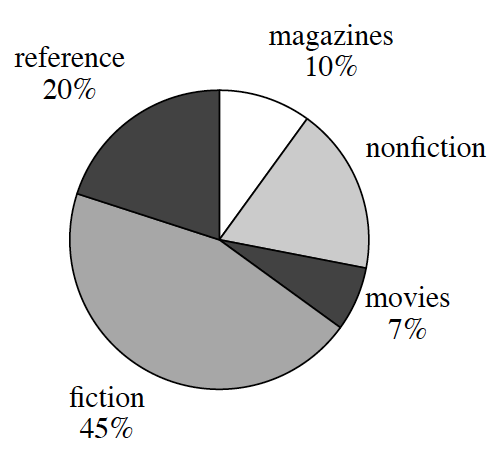### Home > CC3 > Chapter 7 > Lesson 7.1.2 > Problem7-22

7-22.

The school library has $6500$ titles in its collection of books, magazines, and reference materials. The librarian is presenting information about the library to the parent association, and she made the graph below.1. According to the graph, what percent of the collection are nonfiction books?

$100\%-10\%-20\%-45\%-7\%$

$18\%$

2. Could the librarian have presented this information in a histogram? Why or why not?

Recall the qualities of a histogram.
How does it display information?

A histogram displays data as a numerical order.
Since this data is not numerical the librarian cannot present this information in a histogram.

3. How many of the books in the library are fiction?

$\left(\frac{45}{100}\right) \cdot \left(\frac{?}{?}\right) = \frac{\text{answer}}{6500}$